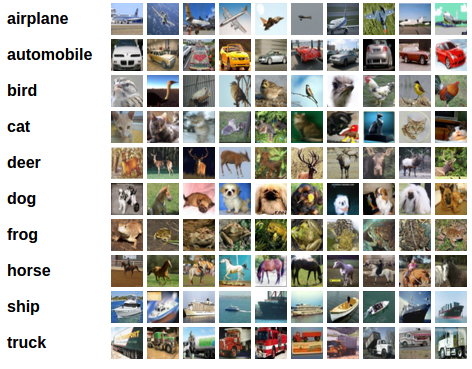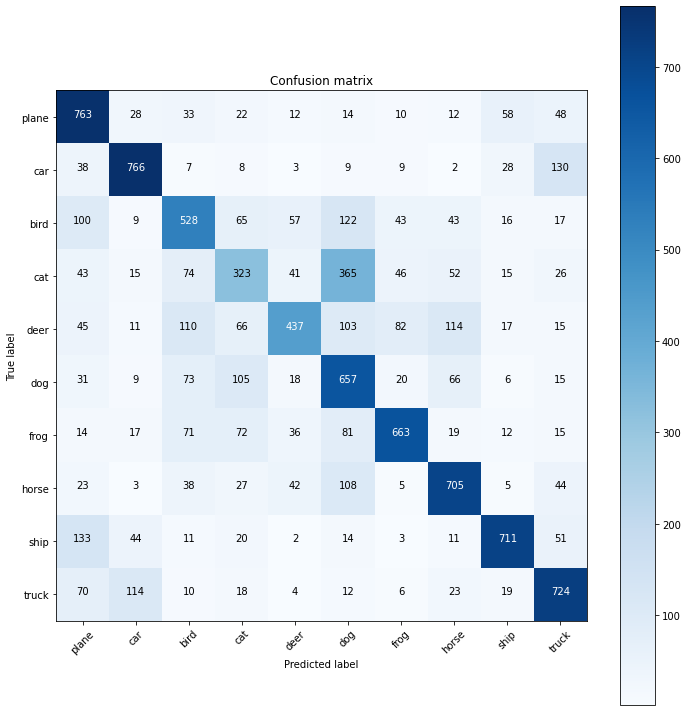In the previous post, we achieved an accuracy around 61%. In this exercise, we will try increasing the accuracy by Hyperparameter Tuning with tensorBoard.

For this exercise, we will use the CIFAR10 dataset. It has the classes: ‘airplane’, ‘automobile’, ‘bird’, ‘cat’, ‘deer’, ‘dog’, ‘frog’, ‘horse’, ‘ship’, ‘truck’. The images in CIFAR-10 are of size 3x32x32, i.e. 3-channel color images of 32x32 pixels in size.The dataset is divided into five training batches and one test batch, each with 10000 images. The test batch contains exactly 1000 randomly-selected images from each class. The training batches contain the remaining images in random order, but some training batches may contain more images from one class than another. Between them, the training batches contain exactly 5000 images from each class.

```pip install tensorboardX
```
```Collecting tensorboardX
|████████████████████████████████| 317kB 2.7MB/s
Requirement already satisfied: numpy in /usr/local/lib/python3.6/dist-packages (from tensorboardX) (1.18.5)
Requirement already satisfied: protobuf>=3.8.0 in /usr/local/lib/python3.6/dist-packages (from tensorboardX) (3.12.4)
Requirement already satisfied: six in /usr/local/lib/python3.6/dist-packages (from tensorboardX) (1.15.0)
Requirement already satisfied: setuptools in /usr/local/lib/python3.6/dist-packages (from protobuf>=3.8.0->tensorboardX) (50.3.0)
Installing collected packages: tensorboardX
Successfully installed tensorboardX-2.1
```
```pip install tensorboard
```
```Requirement already satisfied: tensorboard in /usr/local/lib/python3.6/dist-packages (2.3.0)
Requirement already satisfied: absl-py>=0.4 in /usr/local/lib/python3.6/dist-packages (from tensorboard) (0.10.0)
Requirement already satisfied: grpcio>=1.24.3 in /usr/local/lib/python3.6/dist-packages (from tensorboard) (1.32.0)
Requirement already satisfied: markdown>=2.6.8 in /usr/local/lib/python3.6/dist-packages (from tensorboard) (3.2.2)
Requirement already satisfied: werkzeug>=0.11.15 in /usr/local/lib/python3.6/dist-packages (from tensorboard) (1.0.1)
Requirement already satisfied: tensorboard-plugin-wit>=1.6.0 in /usr/local/lib/python3.6/dist-packages (from tensorboard) (1.7.0)
Requirement already satisfied: requests<3,>=2.21.0 in /usr/local/lib/python3.6/dist-packages (from tensorboard) (2.23.0)
Requirement already satisfied: protobuf>=3.6.0 in /usr/local/lib/python3.6/dist-packages (from tensorboard) (3.12.4)
Requirement already satisfied: setuptools>=41.0.0 in /usr/local/lib/python3.6/dist-packages (from tensorboard) (50.3.0)
Requirement already satisfied: six>=1.10.0 in /usr/local/lib/python3.6/dist-packages (from tensorboard) (1.15.0)
Requirement already satisfied: wheel>=0.26; python_version >= "3" in /usr/local/lib/python3.6/dist-packages (from tensorboard) (0.35.1)
Requirement already satisfied: numpy>=1.12.0 in /usr/local/lib/python3.6/dist-packages (from tensorboard) (1.18.5)
Requirement already satisfied: importlib-metadata; python_version < "3.8" in /usr/local/lib/python3.6/dist-packages (from markdown>=2.6.8->tensorboard) (2.0.0)
Requirement already satisfied: urllib3!=1.25.0,!=1.25.1,<1.26,>=1.21.1 in /usr/local/lib/python3.6/dist-packages (from requests<3,>=2.21.0->tensorboard) (1.24.3)
Requirement already satisfied: chardet<4,>=3.0.2 in /usr/local/lib/python3.6/dist-packages (from requests<3,>=2.21.0->tensorboard) (3.0.4)
Requirement already satisfied: idna<3,>=2.5 in /usr/local/lib/python3.6/dist-packages (from requests<3,>=2.21.0->tensorboard) (2.10)
Requirement already satisfied: certifi>=2017.4.17 in /usr/local/lib/python3.6/dist-packages (from requests<3,>=2.21.0->tensorboard) (2020.6.20)
Requirement already satisfied: rsa<5,>=3.1.4; python_version >= "3" in /usr/local/lib/python3.6/dist-packages (from google-auth<2,>=1.6.3->tensorboard) (4.6)
Requirement already satisfied: zipp>=0.5 in /usr/local/lib/python3.6/dist-packages (from importlib-metadata; python_version < "3.8"->markdown>=2.6.8->tensorboard) (3.2.0)
Requirement already satisfied: pyasn1>=0.1.3 in /usr/local/lib/python3.6/dist-packages (from rsa<5,>=3.1.4; python_version >= "3"->google-auth<2,>=1.6.3->tensorboard) (0.4.8)
```
```import torch
import torch.nn as nn
import torch.optim as optim
import torch.nn.functional as F

import torchvision
import torchvision.transforms as transforms

import numpy as np
import matplotlib.pyplot as plt

from sklearn.metrics import confusion_matrix

from tensorboardX import SummaryWriter
from itertools import product
```
```print(torch.__version__)
print(torchvision.__version__)
```
```1.6.0+cu101
0.7.0+cu101
```

```transform =transforms.Compose([transforms.ToTensor(), transforms.Normalize((0.5, 0.5, 0.5), (0.5, 0.5, 0.5))])

```
```Downloading https://www.cs.toronto.edu/~kriz/cifar-10-python.tar.gz to ./data/cifar-10-python.tar.gz
Extracting ./data/cifar-10-python.tar.gz to ./data
```

## Neural Network and PyTorch design

```def get_num_correct(preds, labels):
return preds.argmax(dim=1).eq(labels).sum().item()
```
```class Network(nn.Module):
def __init__(self):
super(Network,self).__init__()
self.conv1 = nn.Conv2d(in_channels=3, out_channels=6, kernel_size=5)
self.conv2 = nn.Conv2d(in_channels=6, out_channels=16, kernel_size=5)

self.fc1 = nn.Linear(in_features=16*5*5, out_features=120)
self.fc2 = nn.Linear(in_features=120, out_features=84)
self.out = nn.Linear(in_features=84, out_features=10)

def forward(self, t):
#Layer 1
t = t
#Layer 2
t = self.conv1(t)
t = F.relu(t)
t = F.max_pool2d(t, kernel_size=2, stride=2)#output shape : (6,14,14)
#Layer 3
t = self.conv2(t)
t = F.relu(t)
t = F.max_pool2d(t, kernel_size=2, stride=2)#output shape : (16,5,5)
#Layer 4
t = t.reshape(-1, 16*5*5)
t = self.fc1(t)
t = F.relu(t)#output shape : (1,120)
#Layer 5
t = self.fc2(t)
t = F.relu(t)#output shape : (1, 84)
#Layer 6/ Output Layer
t = self.out(t)#output shape : (1,10)

return t
```

## Training the Neural Network

At first, we will experiment with various values of learning rate and batch size. We have also used the Shuffle value to True or false inthis particular case for demonstration. Shuffle for a training data should always be set to `True`.

```parameters = dict(
lr = [.01, .001]
,batch_size = [100, 1000]
,shuffle = [True, False]
)

param_values = [v for v in parameters.values()]
param_values
```
`[[0.01, 0.001], [100, 1000], [True, False]]`
```for lr, batch_size, shuffle in product(*param_values):
print (lr, batch_size, shuffle)
```
```0.01 100 True
0.01 100 False
0.01 1000 True
0.01 1000 False
0.001 100 True
0.001 100 False
0.001 1000 True
0.001 1000 False
```

We will train the network for each combination for 5 epochs.

```for lr, batch_size, shuffle in product(*param_values):
comment = f' batch_size={batch_size} lr={lr} shuffle={shuffle}'
network = Network()
grid = torchvision.utils.make_grid(images)
tb = SummaryWriter(comment=comment)
for epoch in range(5):
total_loss = 0
total_correct = 0
images, labels = batch # Get Batch
preds = network(images) # Pass Batch
loss = F.cross_entropy(preds, labels) # Calculate Loss
optimizer.step() # Update Weights

total_loss += loss.item() * batch_size
total_correct += get_num_correct(preds, labels)

for name, param in network.named_parameters():

print("epoch", epoch, "total_correct:", total_correct, "loss:", total_loss)
tb.close()
```
```epoch 0 total_correct: 18913 loss: 84416.04677438736
epoch 1 total_correct: 22884 loss: 75011.52611970901
epoch 2 total_correct: 24004 loss: 72498.98253679276
epoch 3 total_correct: 24446 loss: 71079.53473329544
epoch 4 total_correct: 25172 loss: 69767.30787754059
epoch 0 total_correct: 18080 loss: 85815.07077217102
epoch 1 total_correct: 22393 loss: 75708.69245529175
epoch 2 total_correct: 23443 loss: 73317.35738515854
epoch 3 total_correct: 24138 loss: 71403.88944149017
epoch 4 total_correct: 24549 loss: 70284.72211360931
epoch 0 total_correct: 16158 loss: 91712.37576007843
epoch 1 total_correct: 23656 loss: 72345.58689594269
epoch 2 total_correct: 26402 loss: 65827.77202129364
epoch 3 total_correct: 27780 loss: 62125.81276893616
epoch 4 total_correct: 29018 loss: 58600.123167037964
epoch 0 total_correct: 13378 loss: 97205.75332641602
epoch 1 total_correct: 21074 loss: 78503.47900390625
epoch 2 total_correct: 24219 loss: 70818.36271286011
epoch 3 total_correct: 25917 loss: 66917.46950149536
epoch 4 total_correct: 27061 loss: 64042.30237007141
epoch 0 total_correct: 18627 loss: 85521.23121023178
epoch 1 total_correct: 24836 loss: 69433.90280008316
epoch 2 total_correct: 27425 loss: 63158.92490148544
epoch 3 total_correct: 29103 loss: 58870.00452876091
epoch 4 total_correct: 30088 loss: 55997.095000743866
epoch 0 total_correct: 18632 loss: 86631.54971599579
epoch 1 total_correct: 24611 loss: 70948.91862869263
epoch 2 total_correct: 27004 loss: 64610.34981012344
epoch 3 total_correct: 28478 loss: 60442.202669382095
epoch 4 total_correct: 29625 loss: 57259.26607251167
epoch 0 total_correct: 12380 loss: 102817.72804260254
epoch 1 total_correct: 18081 loss: 87027.38559246063
epoch 2 total_correct: 20270 loss: 81504.30190563202
epoch 3 total_correct: 21327 loss: 78629.82904911041
epoch 4 total_correct: 22309 loss: 76317.25633144379
epoch 0 total_correct: 12731 loss: 102762.18891143799
epoch 1 total_correct: 18111 loss: 87888.2976770401
epoch 2 total_correct: 20812 loss: 80129.80782985687
epoch 3 total_correct: 22642 loss: 75342.45681762695
epoch 4 total_correct: 24057 loss: 71779.08217906952
```
```%load_ext tensorboard
%tensorboard --logdir runs
```

### Hyperparameter Tuning

• We get the highest accuracy with batch size = 100, learning rate = 0.001.

• We get the least loss with batch size = 100, learning rate = 0.001.

• We get the highest number of correct with batch size = 100, learning rate = 0.001

Next, we will train our model with the above hyperparameters for 20 epochs. The Shuffle should always be set to `True` for a dataset that is to be used for training. for better results, try training with 30-40 epochs.

```network = Network()
for epoch in range(20):

total_correct = 0
total_loss = 0
for batch in train_loader: #Get batch
images, labels = batch #Unpack the batch into images and labels

preds = network(images) #Pass batch
loss = F.cross_entropy(preds, labels) #Calculate Loss

optimizer.step() #Update weights

total_loss += loss.item() * batch_size
total_correct += preds.argmax(dim=1).eq(labels).sum().item()

print('epoch:', epoch, "total_correct:", total_correct, "loss:", total_loss)

print('>>> Training Complete >>>')
```
```epoch: 0 total_correct: 18530 loss: 853069.2903995514
epoch: 1 total_correct: 25004 loss: 694644.5602178574
epoch: 2 total_correct: 27074 loss: 637538.0937457085
epoch: 3 total_correct: 28671 loss: 598220.732986927
epoch: 4 total_correct: 30016 loss: 566515.201985836
epoch: 5 total_correct: 30900 loss: 541954.4064998627
epoch: 6 total_correct: 31552 loss: 521362.08987236023
epoch: 7 total_correct: 32245 loss: 502520.00361680984
epoch: 8 total_correct: 32864 loss: 488691.7136311531
epoch: 9 total_correct: 33429 loss: 472790.4091477394
epoch: 10 total_correct: 33773 loss: 458680.98479509354
epoch: 11 total_correct: 34350 loss: 444449.317753315
epoch: 12 total_correct: 34740 loss: 432666.919529438
epoch: 13 total_correct: 35213 loss: 419870.6297278404
epoch: 14 total_correct: 35589 loss: 407635.0798010826
epoch: 15 total_correct: 36037 loss: 396796.40060663223
epoch: 16 total_correct: 36279 loss: 389014.71281051636
epoch: 17 total_correct: 36739 loss: 377336.72922849655
epoch: 18 total_correct: 37137 loss: 366468.3187007904
epoch: 19 total_correct: 37374 loss: 357310.61974167824
>>> Training Complete >>>
```

## Predictions

We will define a function to get all predictions at once.

```@torch.no_grad()
all_preds = torch.tensor([])
images, labels = batch

preds = model(images)
all_preds = torch.cat((all_preds, preds) ,dim=0)

return all_preds
```
```test_preds = get_all_preds(network, test_loader)
actual_labels = torch.Tensor(test_set.targets)
preds_correct = test_preds.argmax(dim=1).eq(actual_labels).sum().item()

print('total correct:', preds_correct)
print('accuracy:', preds_correct / len(test_set))
```
```total correct: 6277
accuracy: 0.6277
```

The model predicted the label with 63 % accuracy, which is NOT that better than what we predicted without hyper-parameter tuning. Next, we will develop a confusion matrix which will demonstrate, in which particular areas our model is performing poorly.

## Confusion Matrix

```import itertools
import numpy as np
import matplotlib.pyplot as plt

def plot_confusion_matrix(cm, classes,
normalize=False,
title='Confusion matrix',
cmap=plt.cm.Blues):
"""
This function prints and plots the confusion matrix.
Normalization can be applied by setting `normalize=True`.
"""
if normalize:
cm = cm.astype('float') / cm.sum(axis=1)[:, np.newaxis]
print("Normalized confusion matrix")
else:
print('Confusion matrix, without normalization')

print(cm)

plt.imshow(cm, interpolation='nearest', cmap=cmap)
plt.title(title)
plt.colorbar()
tick_marks = np.arange(len(classes))
plt.xticks(tick_marks, classes, rotation=45)
plt.yticks(tick_marks, classes)

fmt = '.2f' if normalize else 'd'
thresh = cm.max() / 2.
for i, j in itertools.product(range(cm.shape), range(cm.shape)):
plt.text(j, i, format(cm[i, j], fmt),
horizontalalignment="center",
color="white" if cm[i, j] > thresh else "black")

plt.tight_layout()
plt.ylabel('True label')
plt.xlabel('Predicted label')
```
```cm = confusion_matrix(test_set.targets, test_preds.argmax(dim=1))
classes = ('plane', 'car', 'bird', 'cat', 'deer', 'dog', 'frog', 'horse', 'ship', 'truck')
plt.figure(figsize=(10,10))
plot_confusion_matrix(cm, classes)
```
```Confusion matrix, without normalization
[[763  28  33  22  12  14  10  12  58  48]
[ 38 766   7   8   3   9   9   2  28 130]
[100   9 528  65  57 122  43  43  16  17]
[ 43  15  74 323  41 365  46  52  15  26]
[ 45  11 110  66 437 103  82 114  17  15]
[ 31   9  73 105  18 657  20  66   6  15]
[ 14  17  71  72  36  81 663  19  12  15]
[ 23   3  38  27  42 108   5 705   5  44]
[133  44  11  20   2  14   3  11 711  51]
[ 70 114  10  18   4  12   6  23  19 724]]
```